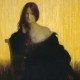## 11 thoughts on “The 2010s Film Alphabet”

1.Slightly Bemused

What I got so far off the top of my head, about half:

A= Arrival
B=
C= Captain America – The First Aevnger
D= Django Unchained
E= Emoji Movie
F=
G=
H=
I= Intersetllar
J= Joker
K= Kickass
L= La La Land
M= Mary Poppins Returns
N=
O=
Q=
R= Revenant
S=
T= True Grit
U=
V= Venom
W=
X=
Y=
Z=

Now to do the research

2.Dermot Hughes

A= Arrival
B=Blackkklansman
C= Captain America – The First Aevnger
D= Django Unchained
E= Emoji Movie
F=Flight
G=Grand Hotel Budapest
H=Her
I= Intersetllar
J= Joker
K= Kickass
L= La La Land
M= Mary Poppins Returns
N=
O=
Q=
R= Revenant
S=Shutter Island
T= True Grit
U=Unbreakable
V= Venom
W=Wolf of Wall St
X=X-Men Days of future past
Y=
Z=Zero Dark Thirty

3.Joxer

B= Black Klansman
F= Flight
X= X-men
z= zombieland ?

1.Slightly Bemused

Darn! Don’t know why I did not get X-Men! I looked at the dark bits, not the blue which is now obviously Mystique :)

4.Slightly Bemused

I forgot one!

S = Shutter Island

5.Jim

N = Nightcrawler

6.Joxer

q= Quartet

7.Solomon Sullivan

A= Arrival
B=BlacKkKlansman
C= Captain America – The First Avenger/Winter Soldier
D= Django Unchained
E= Emoji Movie
F=Flight
G=Grand Budapest Hotel
H=Her
I= Interstellar
J= Joker
K= Kick-Ass
L= LaLa Land
M= Mary Poppins Returns
N=Nightcrawler
O=Other Guys, The
Q=Quartet
R= Revenant
S=Shutter Island
T= True Grit
U=Unbroken
V= Venom
W=Wolf of Wall Street
X=X-Men: Days of Future Past
Z=Zero Dark Thirty

1.Slightly Bemused

Ah, did not see you post before mine, sorry :)

8.Slightly Bemused

Only O and Y to go, so.

I was tempted with O as The Other Guys (does the ‘The’ count?)
Could Y be Your Highness? A film I have never seen, so made no connection

9.Verbatim

Are any of them, apart from Joker, any good?